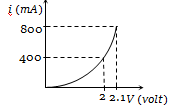NEET Physics Semiconductor Electronics Questions Solved

The i-V characteristic of a P-N junction diode is shown below. The approximate dynamic resistance of the P-N junction when a forward bias of 2 volt is applied(a) 1 $\mathrm{\Omega }$
(b) 0.25 $\mathrm{\Omega }$
(c) 0.5 $\mathrm{\Omega }$
(d) 5 $\mathrm{\Omega }$

Explanation is a part of a Paid Course. To view Explanation Please buy the course.

Difficulty Level: